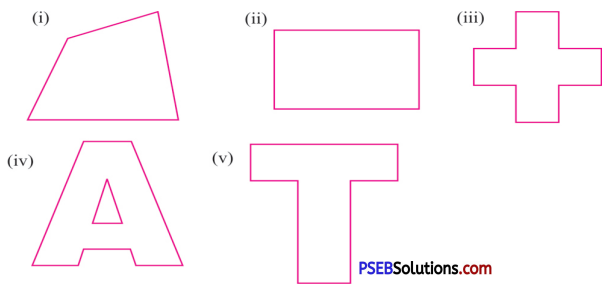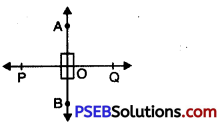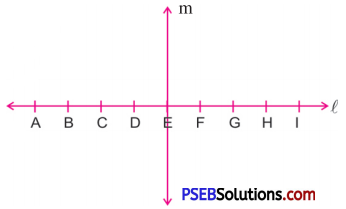# PSEB 6th Class Maths Solutions Chapter 9 Understanding Elementary Shapes Ex 9.3

Punjab State Board PSEB 6th Class Maths Book Solutions Chapter 9 Understanding Elementary Shapes Ex 9.3 Textbook Exercise Questions and Answers.

## PSEB Solutions for Class 6 Maths Chapter 9 Understanding Elementary Shapes Ex 9.3

1. Identify the shape having perpendicular lines:Solution:
(ii), (iii), (v).2. Identify the examples having perpendicular lines:

Question (i)
(i) Lines of railway track.
(ii) Adjacent edges of a table.
(iii) Line segment forming letter ‘L’.
Solution:
(ii) Adjacent edges of a table.
(iii) Line segment forming letter ‘L’.

3. Let $$\overrightarrow{\mathbf{AB}}$$ be perpendicular to $$\overrightarrow{\mathbf{PQ}}$$ and they intersect at O. What is the measure of $$\angle \mathbf{AOP}$$?
Solution:$$\angle \mathbf{AOP}$$ = 90°, because AB ⊥ PQ.4. Line m is perpendicular to line l in the given figure. Each point on the line l is marked at equal intervals. Study the diagram and state true or false.Question (i)
Line m is ⊥ bisector of line segment AI.
Solution:
True

Question (ii)
CE = EG
Solution:
TrueQuestion (iii)
DF = 2DE.
Solution:
True.